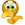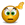# Math - working at basketball shoot - best price, best rate

## Excel Help for Math - Working At Basketball Shoot - Best Price, Best Rate in Charting and Performing Technical Analysis

 Forum Topic Post Reply Login

### Math - Working At Basketball Shoot - Best Price, Best Rate

Rate this:
(3/5 from 1 vote)Math - working at basketball shoot - best price, best rateHello,I wanted to discuss a possible math problem.. perhaps its risk analysis, perhaps its  regression analysis...At a basketball booth, you pay 10 dollars to play, and if you win, you win a 12 dollar prize.My supervisor wants a 10-15% winning rate.The average person is required  to make 4 out of 5 shots rate in order to win the prize. In other words, they must have an an 80% shooting  average.After collecting data, it is determined that most people make somewhere between 2/5 or 3/5 shots, that is 40 to 60 % of their shots..Questions: What is the best required shooting average for a player such that the 10-15% winning rate is met?right now, it seems that 80% required shooting average is too high as very few players have won. Also, based on "RISK", if player can only pay 5 dollars to play, what should the required shooting average be? Should it be 90%?  Thank youJsvlad Posted by jsvlad on 19 Nov 2010
 Replies - Displaying 1 to 3 of 3 Order Replies By: Most Recent | Chronological | Highest RatedRate this:
(3/5 from 1 vote)
This is a simple probability calculation.  In order to have a statistically significant result, we first need to obtain the average and standard deviation of shot rate from the collected data.  For the purposes of this example, we will assume that the average is 60% (3/5) and the standard deviation is 20%.  These can be calculated using the AVERAGE and STDEV functions in Excel.

From this information we can determine the probability of achieving a target shot rate of 80% (4/5). The following spreadsheet and formula in cell B4 illustrates.

Excel Spreadsheet:
 A B 1 Target Rate: 80% 2 Average: 60% 3 Standard Dev: 20% 4 Probability: =1-NORMDIST(B1,B2,B3,TRUE)

The result in cell B4 is 16% (representing the probability of achieving an 80% shoot rate) and this will change based on the average and standard deviation.

In regards to the second question, the target rate should remain unchanged as whether the shooter makes 5 shots or 10 shots, the probability of achieving the target remains unchanged.
Excel Business Forums Administrator
Posted by onRate this: (3/5 from 1 vote) perhaps q2 is not clear..there is more risk for my boss..the customer pays 5 to win 12.. which is a loss of 7 for my bosshe should have a harder task ahead of him..a customer pays 10 to win 10.. which is a loss of 2 for m ybosshe should have an easier task ahead of him.. Posted by jsvlad on 20 Nov 2010Rate this: (3/5 from 1 vote) You can use the same logic to determine the target hit rate for different scenarios. For example, the first scenario assumes that either you gain \$10 or lose \$20.  Plugging this into the probability assumptions the expected payoff is:=(-2*B4)+(10*(1-B4))Where B4 is the cell calculating the probability of winning.  The expected payoff can then be used to calculate the expected profit margin by dividing it by the cost. To model alternative scenarios such as the player (only paying \$5 for a \$12 win) we can simply adjust the target hit rate to obtain the desired hit rate such as 9 out of 10 - 90%, or which ever results in an acceptable expected payoff. Excel Business Forums Administrator Posted by Excel Helper on 20 Nov 2010
 Displaying page 1 of 1

### Excel templates and solutions matched for Math - working at basketball shoot - best price, best rate:

Solutions: Dimensional Analysis and Measurement